SIMCOAL: A general coalescent program for the simulation of molecular data in interconnected populations with arbitrary demography

Introduction

SIMCOAL (ver 1.0) is a computer program for the simulation of molecular genetic diversity in an arbitrary number of haploid populations examined for a set of fully linked loci. It is based on the retrospective coalescent approach initially described by Kingman (1982b; 1982a), and clearly exposed in a series of other papers (Ewens 1990; Hudson 1990; Donnelly and Tavaré 1995). The coalescent backward approach does not simulate the genetic history of the whole population, like in conventional forward simulations, but rather reconstructs the gene genealogy (coalescent history) of samples of genes drawn from different demes in a population. For neutral genes, this coalescent process essentially depends on the history and on the demography of the population, and is independent from the mutational process. In our program, we simulate mutations starting from the most recent common ancestor (MRCA) of all genes in the sample, and add them independently on all branches of the genealogy assuming a uniform and constant Poisson process. Using this two-step (coalescent-mutation) approach, many replicates of haploid samples of DNA sequences, RFLP, or microsatellite data can be simulated very quickly. The analysis of a large number of simulated samples allows one to obtain the empirical distribution of practically any statistic that can be derived from genetic data, including statistics for which no analytical derivation is available (Hudson 1993). Typical applications of our program include the study of the effect of complex demographies on the pattern of genetic diversity within and between populations, like in the case of bottlenecks, complex cases of admixture, or metapopulation systems. While our program generates haploid samples of genes or haplotypes, diploid data can be generated under the hypothesis of Hardy-Weinberg equilibrium by taking random pairs of haplotypes to form diploid genotypes. The program runs on compatible PC computers under Windows 9X/NT, but we provide the C++ source code that should compile under other operating systems, provided the compiler follows the latest C++ ISO committee specifications.

Output files

The final state of each gene is recorded and output to several files according to two data formats. The first format is compatible with the Arlequin program (Schneider et al. 2000). The data generated after each simulation are output to a separate Arlequin project file. An Arlequin batch file is also created, listing all simulated files, and allowing one to compute different statistics on the whole set of simulated files with Arlequin, and thus to obtain their distribution after extraction from the Arlequin output files. Note that with version 2.0 of Arlequin, a utility is provided to collect most statistics computed in the analysis of batch files under the form of MS-Excel compatible files. Examples of statistics that can be extracted from batch file analysis by Arlequin are: nucleotide composition, gene diversity, homozygosity, mean number of pairwise differences, number of haplotypes, number of polymorphic sites, Tajimas D, extent of linkage disequilibrium among loci, mutation parameter q = 2Nu estimated from homozygosity, mean number of pairwise differences, number of polymorphic sites, or number of alleles, and genetic distances based on pairwise FSTs. Note also that the Arlequin software has a file conversion utility for exporting input data files into several other format like Biosys, Phylip, or GenePop, so that files produced by SIMCOAL could be also analyzed by these softwares after file conversion. The two other sets of output file produced by SIMCOAL are compatible with the NEXUS file format. Two files with the *.trees extension list all the simulated trees, with branch lengths expressed either 1) in units of generations scaled by the population size (N), and therefore representing the true coalescent history of the sample of genes, or 2) in units of average number of substitutions per site, and therefore representing the realized mutational tree. These two sets of trees can be visualized with the software TREEVIEW (Page 1996). Finally, a file with the *.paup extension, lists all the simulated genes together with their true genealogical structure. This file can be analyzed with David Swofford's PAUP* software (1999)

Mutation models

RFLP data: Only a pure 2-allele model is implemented. Several fully linked RFLP loci can be simulated, assuming a homogeneous mutational process over all loci. A finite-sites model is used, and mutations can hit the same site several times, switching the RFLP site on and off. We thus assume that there is the same probability for a site loss or for a site gain.

Microsatellite data: We have implemented a pure stepwise mutation model (SMM) with or without constraint on the total size of the microsatellite. Several fully linked microsatellite loci can be simulated under the same mutation model constraints. The output for each loci is listed as a number of repeat, having started arbitrarily at 10,000 repeats. The number of repeats for each gene should thus be centered around that value.

DNA sequence data: We have implemented here several simple finite-sites mutational models. The user can specify the percentage of substitutions that are transitions (the transition bias), the amount of heterogeneity in mutation rates along a DNA sequence according to either a discrete or continuous Gamma distribution. We can therefore simulate DNA sequences under a Jukes and Cantor model (Jukes and Cantor 1969) or under a Kimura-2-parameter model (Kimura 1980), with or without Gamma correction for heterogeneity of mutation rates (Jin and Nei 1990). Other mutation models that depend on the nucleotide composition of the sequence were not considered here, because of their complexity and because they require specifying many additional parameters, like the mutation transition matrix and the equilibrium nucleotide composition.

Demographic models

There is no limitation on sample sizes, deme sizes or number of simulated demes, other than the available memory in the computer and the computational time. Deme sizes should nevertheless always be much larger than the sample sizes for the single coalescent event per generation assumption to hold. Each sample of gene is assumed to be drawn from a different deme that can exchange an arbitrary number of migrants with other demes at any generation. As the migration rates are specified by a migration matrix of size equal to the number of simulated demes, either an island model, a one-dimensional or two dimensional stepping-stone models can be simulated, with demes arranged on flat-surface, a cylinder or a torus. Several migration matrices can be specified in the input file, so that any matrix can be used at any time in the simulation process. The history of several completely separated demes can also be simulated, as in the case of a series of population fissions or admixtures. Historical events can be defined to specify which parental populations are used to produce daughter populations after a fission event, as well as its timing. Note that the program can also be used to simulate the genetic divergence of different species, with or without bottlenecks.

The size of each deme can either remain stationary over time or to change exponentially, separately in each population. Positive or negative exponential growths can be modeled. Historical events can be used to instantaneously modify the size of a deme or to alter its exponential growth pattern at any generation in the past.

Because the coalescent approach is a retrospective approach that goes backward in time, one should be careful that what one considers as population fissions under a forward approach correspond here to population fusions under this approach. Similarly, a period of population growth corresponds to a population decline as seen from the present time. It should thus be clear that even we use either a forward-oriented or backward-oriented description of a given process, the coalescent simulations always proceed backward in time.

Input file

A single input file is needed for the simulation. It should specify the number of gene samples to simulate, the sample sizes, the deme sizes, the exponential growth rate of each deme, the number of linked loci to simulate, and the overall mutation rate for all simulated loci. We can also list a series of historical events that can be used to: a) resize a deme; b) implement an episode of migration from a source to a sink deme (for instance to simulate a population splitting into two sub-populations); c) reset the exponential growth rate of a given deme; or d) switch from a given migration matrix to another one. Note that several historical events can happen at the same generation. The data type (RFLP, microsatellite or DNA) must also be specified in the input file. For DNA data, the proportion of substitutions being transitions must be specified to set a given transition bias, as well as the amount of rate heterogeneity among sites, which can be done by setting the alpha parameter of a gamma distribution. For microsatellite data, the potential size range constraint must also be provided.

Input file notation

Comments: Comments begin with a double slash. They have to be input on a single line in the input file. Comment lines are compulsory, but can be empty (no comment on a blank line)

Demes sizes: Demes can have different sizes.

Samples sizes: Sample sizes can also be unequal among demes. Samples of size zero can also be used.

Growth rate: This is the exponential growth parameter r in N(t) = N(0) exp(rt). Negative growth implies population expansion, because we simulate backward in time.

No. of migration matrices:

Several matrices can be listed in the input file. Their number is specified here. A value of zero means that no migration matrix is provided, and the program will assume that there is no migration between demes.
Migration matrices: A matrix of size equal to the number of listed demes. If no matrix is defined, SIMCOAL creates a migration matrix (with id "0") filled with zeroes, which assumes no migration among demes. The matrices have associated ids that correspond to their order of appearance in the list, starting at zero. If no particular migration matrix is specified with an historical event at generation zero, the matrix "0" (zero) is used.

Historical events: One first lists how many of these events has to be read. A value of zero is valid.
Then, on separate line, one enters each historical event in turn. They are structured as follows:
 1st number: The number of generation since the present generation at which the historical event occured
 2nd number: The source deme id (deme ids are arbitrarily numbered starting at zero)
 3rd number: The sink deme id. Source and sink refer to demes exchanging migrants
 4th number: The proportion of migrants sent from the source deme to the sink deme. It also represents the probability for each lineage remaining in the source deme to migrate in the sink deme.
 5th number: The relative new size of the sink deme: a number smaller than 1 will simulate a population expansion, while a number larger than one will simulate a population constriction  (when looking forward in time).
 6th number: The new growth pattern of the sink deme. Zero means a stationary population.
 7th number: The id of the migration matrix to use. This modification applies to all demes simultaneously.
Mutation rate: This number refers to the mutation rates for all loci taken as a whole. For DNA sequence, it should correspond to the average mutation rate per nucleotides times the number of simulated nucleotides.

Number of loci: Number of completely linked loci to simulate (no recombination is assumed). For DNA, it should be the length of the sequence to simulate.

Data type: Type of data to simulate: Currently microsatellite data, DNA sequence or RFLP microsatellite.
A pure stepwise mutation model is used for microsatellite data, with possible range constraints. Different mutation models are possible for DNA sequences. A simple two alele model is used for RFLP data.

Gamma shape parameter: The first number on this line should be the shape parameter a  of a Gamma distribution of mutation rates. If a value of zero is entered, then an even mutation rate model is implemented. The second number is the number of rate classes to simulate. If a value of zero is entered, then a continuous distribution is used (as many classes as there are loci (or nucleotides).

Examples of input files

1) Simulation of the simultaneous divergence of 3 samples from a single ancestral population

In this example, the populations have unequal size, one being five times larger than the others. the samples sizes are also unequal. The haploid populations are demographically  stationary. Two migration matrices are given. The first migration matrix defines a linear stepping-stone model. The second one specifies an absence of migrations between the populations. Two historical events are implemented to specify the divergence of a single ancestral population into the three populations that we observe nowadays. The size of the ancestral population is equal to the size of the largest derived population. As for the mutation model, we simulate the diversity of DNA sequences of 300 bp, with 10 times more transitions than transversion, and we asssume a discrete gamma distribution of mutation rates with alpha=0.4 and 10 different rate classes.

Graphically, the demography  of the three populations can be represented as follows: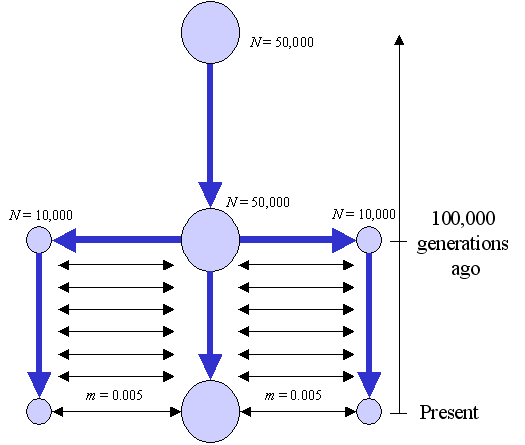File 3sampmig.par

 //Input parameters for the coalescence simulation program : simcoal.exe 3 samples to simulate //Deme sizes (haploid number of genes) 10000 50000 10000 //Sample sizes 20 30 10 //Growth rates 0 0 0 //Number of migration matrices : If 0 : No migration between demes 2 //Migration rates matrix 0 : A linear stepping-stone model 0.000 0.005 0.000 0.005 0.000 0.005 0.000 0.005 0.000 //Migration rates matrix 1 : No migrations 0 0 0 0 0 0 0 0 0 //Historical event: time, source, sink, migrants, new deme size, new growth rate, new migration matrix 2 historical events 100000 0 1 1 1 0 1 100000 2 1 1 1 0 1 //Mutation rate per generation for the whole sequence 0.0005 //Number of loci 300 //Data type : either DNA, RFLP, or MICROSAT : If DNA, second term is the transition bias DNA 0.9090909 //Mutation rates gamma distribution shape parameter 0.4 10 [EOF]

2) An applied example: Testing the hypothesis that two samples have a comparable amount of diversity

Problem: Two samples are examined for their DNA sequence diversity for 300 bp. 20 sequences examined in the first sample reveal 32 polymorphic sites, whereas 10 sequences examined for the second sample reveal only 14 polymorphic sites. One would like to know if the level of diversity found in the second sample is compatible with that found in the first sample, taking into account the sample size difference.

From the diversity pattern of the first sample, we can estimate the mutation parameter q of the population from Watterson (1975) equation relating the expected number of polymorphic sites S the mutation parameter q and the sample size n.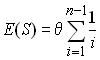We therefore take as an estimator of q the quantity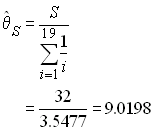The quantity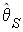estimates the parameter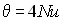. We can fix the population size to 10,000 diploid individuals in order to get an estimate of the mutation rate as 2.25494 10-4

File 1samp14S.par

 //File 1samp14S.par - Input parameters for the coalescence simulation program : simcoal.exe 1 sample to simulate //Deme sizes (haploid number of genes = 2 X the diploid deme size) 20000 //Sample sizes 10 //Growth rates 0 //Number of migration matrices : If 0 : No migration between demes 0 //Historical event: time, source, sink, migrants, new deme size, new growth rate, new migration matrix 0 historical events //Mutation rate per generation for the whole sequence 0.000225494 //Sequence length 300 //Data type : either DNA, RFLP, or MICROSAT : If DNA, second term is the transition bias DNA 0.333333333 //Mutation rates gamma distribution shape parameter 0 0 [EOF]

After an analysis of 1,000 simulated samples, using the Arlequin program, we get the following distribution of the number of polymorphic sites.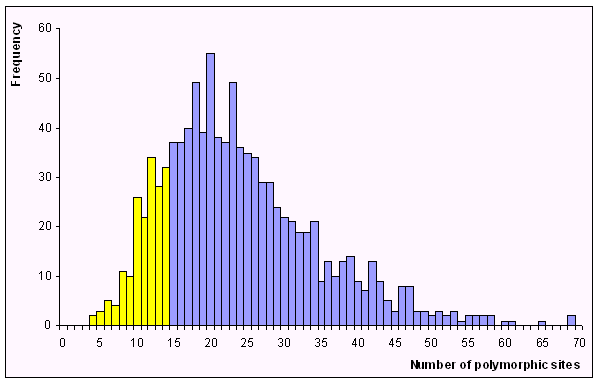We estimate the probability of observing 14 or fewer sites in a sample of 10 sequences to be equal to 0.177. Therefore the two samples are not statistically different for the number of polymorphic sites.

Other examples of input files

 testmicro1.par : 2 populations, no migration, microsatellite data with mutation range constraint for the stepwise mutation model
 testmicro2.par : 2 populations, no migration, microsatellite data, no mutation range constraint for the stepwise mutation model
 testdna1.par : 2 populations, no migration, DNA sequence data, transition bias, continuous gamma distribution of mutation rates
 testdna2.par : 2 populations, no migration, DNA sequence data, transition bias, discrete gamma distribution of mutation rates (8 classes)
 4popisland.par : 4 populations,DNA sequence data, transition bias, even mutation rates among sites, two migration matrices, 3 historical events to implement a series of population fusions at different times, we switch from an island migration model to no migration when the first population fusion begins. 20popisland.par : 4 populations,DNA sequence data, transition bias, even mutation rates among sites, two migration matrices, 3 historical events to implement a series of population fusions at different times, we switch from an island migration model to no migration when the first population fusion begins.

Running simcoal

There are three ways to run simcoal:

1. simcoal <no arguments>
The program will prompt you for :
a) an input file name to process (just enter the name of the file without the ".par" extension )
b) the number of simulations to perform.

2. simcoal <batch file name>
The program will open the batch file that should contain, on the first
line, the number of simulations to perform, and on the following lines
the names of the input files to process
Example: simcoal testbatch.txt

3. sim_coal <file name> <number of simulations to perform>
Example: simcoal testmicro1.par 1000

References

• Donnelly P, and Tavaré S, 1995. Coalescents and genealogical structure under neutrality. Annu. Rev. Genet. 29:401-421.

• Ewens WJ, 1990. Population Genetics Theory - The Past and the Future. In: Mathematical and Statistical developments of Evolutionary Theory, (Lessar S, eds.), Dordrecht: Kluwer Academic Publishers; 177-227.

• Hudson RR, 1990. Gene genealogies and the coalescent proces. In: Oxford Surveys in Evolutionary Biology, (Futuyma DJ, and Antonovics JD, eds.), New York: Oxford University Press; 1-44.

• Hudson RR, 1993. The how and why of generating gene genealogies. In: Mechanisms of Molecular Evolution, (Takahata N, and Clark AG, eds.), Sunderland: Sinauer Ass. Inc.; 23-36.

• Jin L, and Nei M, 1990. Limitations of the evolutionary parsimony method of phylogenetic analysis. Mol. Biol. Evol. 7:82-102.

• Kingman JFC, 1982a. The coalescent. Stoch. Proc. Appl. 13:235-248.

• Kingman JFC, 1982b. On the genealogy of large populations. J. Appl. Proba. 19A:27-43.

• Page RDM, 1996. TREEVIEW: An application to display phylogenetic trees on personal computers. Comput. Appl. Biosci. 12:357-358.

• Schneider S, Roessli D and Excoffier L, 2000. Arlequin: a software for population genetics data analysis. User manual ver 2.000. Ver. 2.000. Geneva: Genetics and Biometry Lab, Dept. of Anthropology, University of Geneva.
http://anthro.unige.ch/arlequin

• Watterson G, 1975. On the number of segregating sites in genetical models without recombination. Theor.Popul.Biol. 7:256-276.

Laurent Excoffier, Computational and Molecular Population Genetics Lab, University of Bern

Last edited on 14.10.03 (08:26)